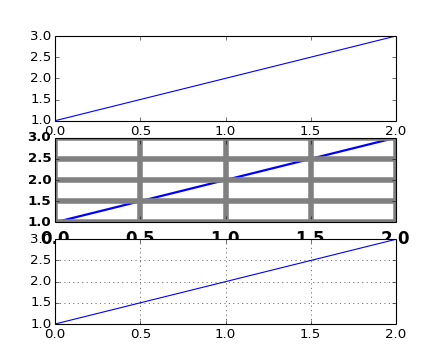# pylab_examples example code: customize_rc.py¶```"""
I'm not trying to make a good looking figure here, but just to show
some examples of customizing rc params on the fly

If you like to work interactively, and need to create different sets
of defaults for figures (eg one set of defaults for publication, one
set for interactive exploration), you may want to define some
functions in a custom module that set the defaults, eg

def set_pub():
rc('font', weight='bold')    # bold fonts are easier to see
rc('tick', labelsize=15)     # tick labels bigger
rc('lines', lw=1, color='k') # thicker black lines (no budget for color!)
rc('grid', c='0.5', ls='-', lw=0.5)  # solid gray grid lines
rc('savefig', dpi=300)       # higher res outputs

Then as you are working interactively, you just need to do

>>> set_pub()
>>> subplot(111)
>>> plot([1,2,3])
>>> savefig('myfig')
>>> rcdefaults()  # restore the defaults

"""
from pylab import *

subplot(311)
plot([1,2,3])

# the axes attributes need to be set before the call to subplot
rc('font', weight='bold')
rc('xtick', labelsize=15)

# using aliases for color, linestyle and linewidth; gray, solid, thick
rc('grid', c='0.5', ls='-', lw=5)
rc('lines', lw=2, color='g')
subplot(312)

plot([1,2,3])
grid(True)

rcdefaults()
subplot(313)
plot([1,2,3])
grid(True)
show()
```

Keywords: python, matplotlib, pylab, example, codex (see Search examples)